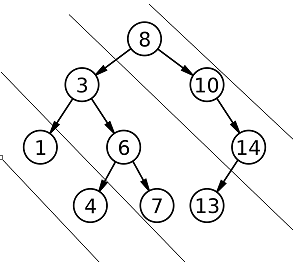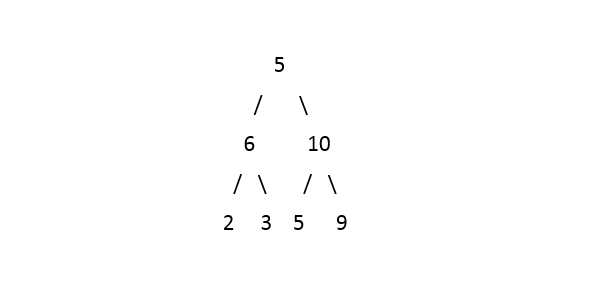# Diagonal Sum

Posted: 9 Oct, 2020
Difficulty: Easy

## PROBLEM STATEMENT

#### Diagonals of the binary tree are :#### Diagonal 3 : 1 4

``````Example :

For the given binary tree :
````````````Output : 24 14 2

Explanation: Rightmost diagonal contains elements {5, 10 , 9} its sum is 24, middle diagonal contains elements {6, 3, 5} its sum is 14, leftmost diagonal contains elements {2}. Thus the answer should be “24 14 2”.
``````
##### Input Format :
``````The only line of the test case contains elements in the level order form. The line consists of values of nodes separated by a single space. In case a node is null, we take -1 on its place.

For example, the input for the tree depicted in the below image would be :
````````````1
2 3
4 -1 5 6
-1 7 -1 -1 -1 -1
-1 -1
``````

#### Explanation :

``````Level 1 :
The root node of the tree is 1

Level 2 :
Left child of 1 = 2
Right child of 1 = 3

Level 3 :
Left child of 2 = 4
Right child of 2 = null (-1)
Left child of 3 = 5
Right child of 3 = 6

Level 4 :
Left child of 4 = null (-1)
Right child of 4 = 7
Left child of 5 = null (-1)
Right child of 5 = null (-1)
Left child of 6 = null (-1)
Right child of 6 = null (-1)

Level 5 :
Left child of 7 = null (-1)
Right child of 7 = null (-1)

The first not-null node (of the previous level) is treated as the parent of the first two nodes of the current level. The second not-null node (of the previous level) is treated as the parent node for the next two nodes of the current level and so on.
The input ends when all nodes at the last level are null (-1).
``````
##### Note :
``````The above format was just to provide clarity on how the input is formed for a given tree.
The sequence will be put together in a single line separated by a single space. Hence, for the above-depicted tree, the input will be given as:

1 2 3 4 -1 5 6 -1 7 -1 -1 -1 -1 -1 -1
``````
##### Output Format :
``````Print the only line of output will contain a list containing diagonal sums from right diagonal to left diagonal.
``````
##### Note :
``````You are not required to print the output explicitly, it has already been taken care of. Just implement the function.
``````
##### Constraints :
``````0 <= N <= 10^5
0 <= node.data <= 10^9

Time Limit : 1 sec
``````Approach 1

1. To store diagonal sum for each diagonal separately, HashMap (say, ‘MAP’) is used with its key as diagonal number and value as the sum of nodes in that diagonal.
2. Assign a distance 0 to the root.
3. Create a static variable ‘maxSlope’ that will store the maximum slope of the tree i.e total no of diagonal.
4. Run a helper function with parameter ‘ROOT’, distance as 0 and 'MAP'.
5. In the helper function,
• Check if the ‘ROOT’ is ‘NULL’, return.
• Update the ‘maxSlope’ to maximum of ‘maxSlope’ and ‘D’.
• Update the diagonal sum in the ‘MAP’.
• Recur for left by incrementing ‘D’ by 1.
• Recur for right but do not change ‘D’.
6. Finally, Initialise a list for storing the diagonal sum.
7. Run a loop from 0 to ‘maxSlope’ and for each diagonal add the sum in the list and return the list.